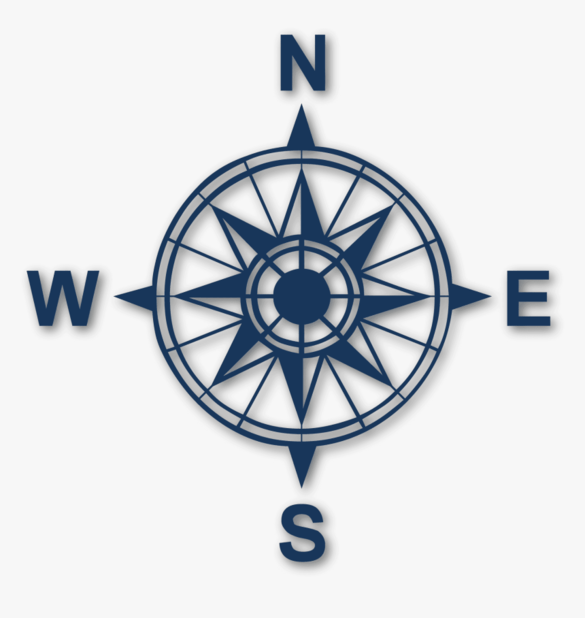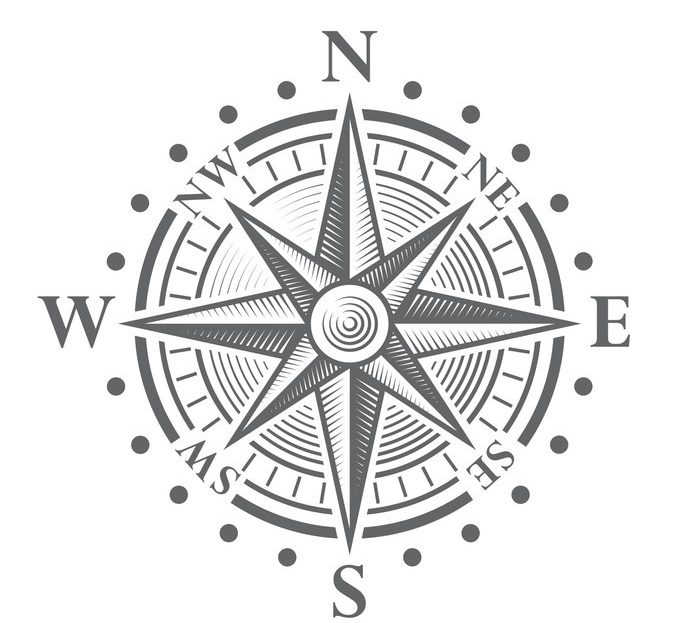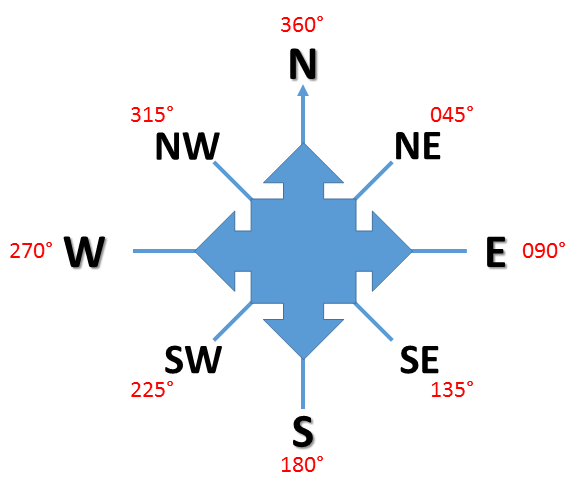# BEARING

Welcome to Class !!

In today’s Mathematics class, We will be talking about Bearing. We hope you enjoy the class!

## BEARING

CONTENT:

i)   Compass bearing

ii) Three figure bearing

iii)  Finding the bearing of a point from another.

### Compass Bearing

A bearing gives the direction between two points in terms of an angle in degrees. The two types of bearings are compass bearings and three-figure bearings.

The four major compass directions are North (N), South (S), East (E), and West (W).N

W                                              E

S

In compass bearings, the angles are measured from North or South depending on which one is nearer

NE         N            NE

W                                              E

SW               S           SE

Apart from the four main points or directions, there are also four main secondary directions i.e. NE (North East), SE (South East), SW (South West), NW (North West). The angle between each point is 450.Worked Examples

Draw a sketch to show each of these bearings marking the angles clearly.

1. (a) N 350 W   (b) N 700 E   (c) S 580

Solution

1. a) N 350 W means from N, measures 350 towards the W or 350 W of N.

N 350 W            N

1. b) N 700 E means 700 towards E.

N

N 700 E

W                        E

1. c) S 580W means from S measures 580 towards W.

N

W                                         E

S

S580W

Evaluation:

Find the compass direction of point A from point O in these diagrams.

1. a) N b)

N

W                                     E                            W                                      E

S                                                                     S

1. c) N

W                                     E

S

REFERENCE

NGM Bk 2 Chapter 23, Page 185 – 187.

Essential Mathematics for JSS Bk 2, Chapter 24, Pg 246 – 247.

THREE FIGURE BEARINGS

Three figure bearings are given as the number of degrees from North, measured in a clockwise direction. Any direction can be given as a three-figure bearing. Three digits are always given but for angles less than 1000 an extra zero need to be written in front of the digits e.g. 0000, 0360, 0700 up to 0990.Worked Examples

Find the three-figure bearings of A, B, C, and D from X.

N           A

D

W                          X                            E

C

S        B

Solution

a) The arrow N shows the direction N, NXA = 630. The bearing of A from X is 0630.

b) NXB = 180 – 35 = 1450. The bearing of B from X is 1450.

c) NXC clockwise = 180 + 75 = 2550. The bearing of C from X is 2550.

d) NXD clockwise = 360 – 52 = 3080. The bearing of D from X is 3080.

We have come to the end of this class. We do hope you enjoyed the class?

Should you have any further question, feel free to ask in the comment section below and trust us to respond as soon as possible.

In our next class, we will be talking about Scale Drawing. We are very much eager to meet you there.

Are you a Parent? Share your quick opinion and win free 2-month Premium Subscription

### 2 thoughts on “BEARING”

1.Seriously, can’t you upgrade the way u demonstrate?

1.i know right?

Don`t copy text!